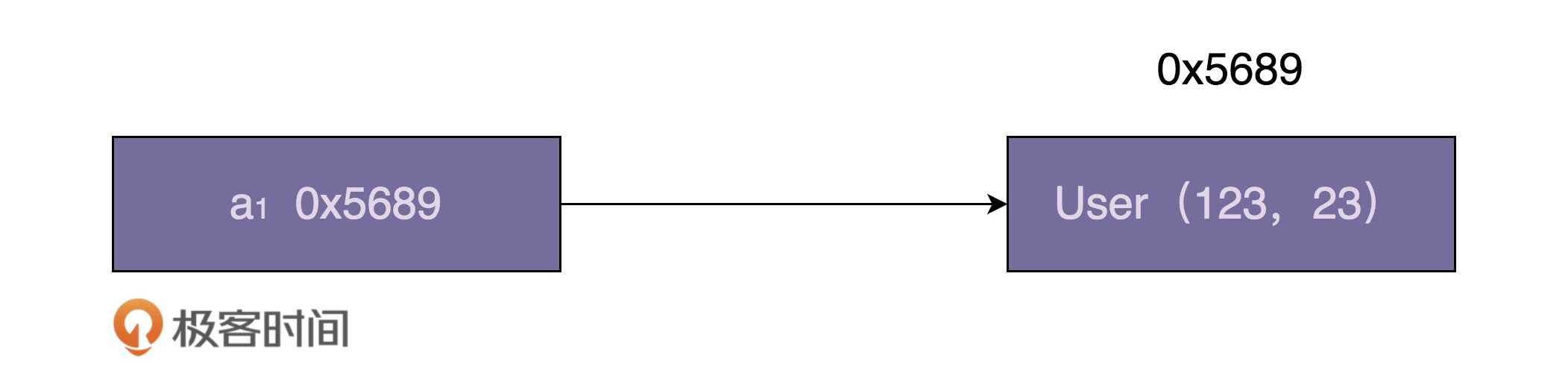## 享元模式在 Java Integer 中的应用

Integer i1 = 56;
Integer i2 = 56;
Integer i3 = 129;
Integer i4 = 129;
System.out.println(i1 == i2);
System.out.println(i3 == i4);

• 如何判定两个 Java 对象是否相等（也就代码中的“==”操作符的含义）？
• 什么是自动装箱（Autoboxing）和自动拆箱（Unboxing）？

//自动装箱
Integer i = 56;
//自动拆箱
int j = i;

Integer i = 59；底层执行了：Integer i = Integer.valueOf(59);

int j = i; 底层执行了：int j = i.intValue();

User a = new User(123, 23); // id=123, age=23Integer i1 = 56;
Integer i2 = 56;
Integer i3 = 129;
Integer i4 = 129;
System.out.println(i1 == i2);
System.out.println(i3 == i4);

4 行赋值语句都会触发自动装箱操作，也就是会创建 Integer 对象并且赋值给 i1、i2、i3、i4 这四个变量。根据刚刚的讲解，i1、i2 尽管存储的数值相同，都是 56，但是指向不同的 Integer 对象，所以通过“==”来判定是否相同的时候，会返回 false。同理，i3==i4 判定语句也会返回 false

public static Integer valueOf(int i) {
if (i >= IntegerCache.low && i <= IntegerCache.high)
return IntegerCache.cache[i + (-IntegerCache.low)];
return new Integer(i);
}

/**
* Cache to support the object identity semantics of autoboxing for values between
* -128 and 127 (inclusive) as required by JLS.
*
* The cache is initialized on first usage.  The size of the cache
* may be controlled by the {@code -XX:AutoBoxCacheMax=<size>} option.
* During VM initialization, java.lang.Integer.IntegerCache.high property
* may be set and saved in the private system properties in the
* sun.misc.VM class.
*/
private static class IntegerCache {
static final int low = -128;
static final int high;
static final Integer cache[];

static {
// high value may be configured by property
int h = 127;
String integerCacheHighPropValue =
sun.misc.VM.getSavedProperty("java.lang.Integer.IntegerCache.high");
if (integerCacheHighPropValue != null) {
try {
int i = parseInt(integerCacheHighPropValue);
i = Math.max(i, 127);
// Maximum array size is Integer.MAX_VALUE
h = Math.min(i, Integer.MAX_VALUE - (-low) -1);
} catch( NumberFormatException nfe) {
// If the property cannot be parsed into an int, ignore it.
}
}
high = h;

cache = new Integer[(high - low) + 1];
int j = low;
for(int k = 0; k < cache.length; k++)
cache[k] = new Integer(j++);

// range [-128, 127] must be interned (JLS7 5.1.7)
assert IntegerCache.high >= 127;
}

private IntegerCache() {}
}

IntegerCache 的代码实现中，当这个类被加载的时候，缓存的享元对象会被集中一次性创建好。毕竟整型值太多了，我们不可能在 IntegerCache 类中预先创建好所有的整型值，这样既占用太多内存，也使得加载 IntegerCache 类的时间过长。所以，我们只能选择缓存对于大部分应用来说最常用的整型值，也就是一个字节的大小（-128127 之间的数据）。

//方法一：
-Djava.lang.Integer.IntegerCache.high=255
//方法二：
-XX:AutoBoxCacheMax=255

private static class LongCache {
private LongCache(){}

static final Long cache[] = new Long[-(-128) + 127 + 1];

static {
for(int i = 0; i < cache.length; i++)
cache[i] = new Long(i - 128);
}
}

public static Long valueOf(long l) {
final int offset = 128;
if (l >= -128 && l <= 127) { // will cache
return LongCache.cache[(int)l + offset];
}
return new Long(l);
}

Integer a = new Integer(123);
Integer a = 123;
Integer a = Integer.valueOf(123);

## 享元模式在 Java String 中的应用

Integer 类的设计思路相似，String 类利用享元模式来复用相同的字符串常量。JVM 会专门开辟一块存储区来存储字符串常量，这块存储区叫作“字符串常量池”。

## 重点

Java Integer 的实现中，-128127 之间的整型对象会被事先创建好，缓存在 IntegerCache 类中。当我们使用自动装箱或者 valueOf() 来创建这个数值区间的整型对象时，会复用 IntegerCache 类事先创建好的对象。这里的 IntegerCache 类就是享元工厂类，事先创建好的整型对象就是享元对象。

Java String 类的实现中，JVM 开辟一块存储区专门存储字符串常量，这块存储区叫作字符串常量池，类似于 Integer 中的 IntegerCache。不过，跟 IntegerCache 不同的是，它并非事先创建好需要共享的对象，而是在程序的运行期间，根据需要来创建和缓存字符串常量。